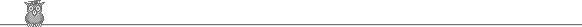### IMO 1979Problem A1

Let m and n be positive integers such that:

m/m = 1 - 1/2 + 1/3 - 1/4 + ... - 1/1318 + 1/1319.

Prove that m is divisible by 1979.

Solution

This is difficult.

The obvious step of combining adjacent terms to give 1/(n(n+1) is unhelpful. The trick is to separate out the negative terms:

1 - 1/2 + 1/3 - 1/4 + ... - 1/1318 + 1/1319 = 1 + 1/2 + 1/3 + ... + 1/1319 - 2(1/2 + 1/4 + ... + 1/1318) = 1/660 + 1/661 + ... + 1/1319.

and to notice that 660 + 1319 = 1979. Combine terms in pairs from the outside:

1/660 + 1/1319 = 1979/(660.1319); 1/661 + 1/1318 = 1979/(661.1318) etc.

There are an even number of terms, so this gives us a sum of terms 1979/m with m not divisible by 1979 (since 1979 is prime and so does not divide any product of smaller numbers). Hence the sum of the 1/m gives a rational number with denominator not divisible by 1979 and we are done.Solutions are also available in     Murray S Klamkin, International Mathematical Olympiads 1978-1985, MAA 1986, and in   István Reiman, International Mathematical Olympiad 1959-1999, ISBN 189-8855-48-X.